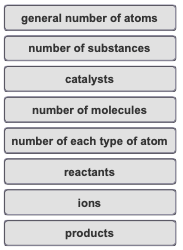# Problem: What is a balanced chemical equation?Match the words and phrases in the left column to the appropriate blanks in the sentences on the right.In chemistry, we represent chemical reactions with chemical equations where the substances on the left-hand side of a chemical equation are the _________ and the substances on the right-hand side are the ____________. Chemical equations are balanced when the _____________ on the left side of the equation is equal to the number on the right side.

###### FREE Expert Solution

balanced chemical equation → same number of atoms in the reactant and product side

81% (388 ratings)###### Problem Details

What is a balanced chemical equation?

Match the words and phrases in the left column to the appropriate blanks in the sentences on the right.

In chemistry, we represent chemical reactions with chemical equations where the substances on the left-hand side of a chemical equation are the _________ and the substances on the right-hand side are the ____________. Chemical equations are balanced when the _____________ on the left side of the equation is equal to the number on the right side.